NCERT Solutions: Statistics (Exercise 12.1)

# Statistics (Exercise 12.1) NCERT Solutions - Mathematics (Maths) Class 9

Q1. A survey conducted by an organisation for the cause of illness and death among the women between the ages 15–44 (in years) worldwide, found the following figures (in %):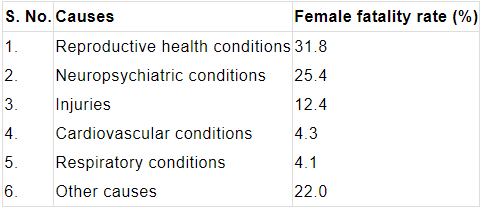(i) Represent the information given above graphically.
(ii) Which condition is the major cause of women’s ill health and death worldwide?
(iii) Try to find out, with the help of your teacher, any two factors which play a major role in the cause in (ii) above being the major cause.
Ans:
(i) The information given in the question is represented below graphically.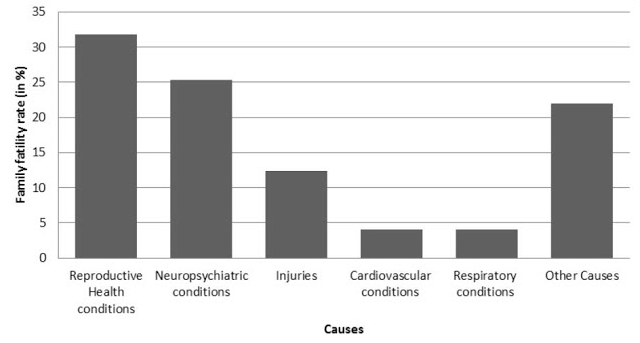(ii) We can observe from the graph that reproductive health conditions is the major cause of women’s ill health and death worldwide.
(iii) Two factors responsible for cause in (ii) are:

• Lack of proper care and understanding.
• Lack of medical facilities.

Q2. The following data on the number of girls (to the nearest ten) per thousand boys in different sections of Indian society is given below.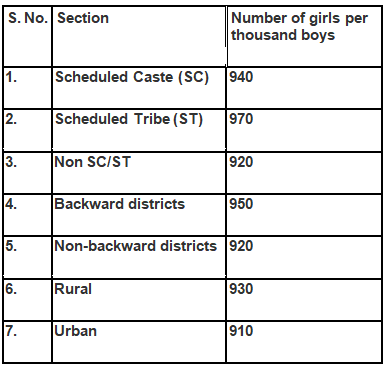(i) Represent the above information by a bar graph.
Ans: (i) The information given in the question is represented below graphically.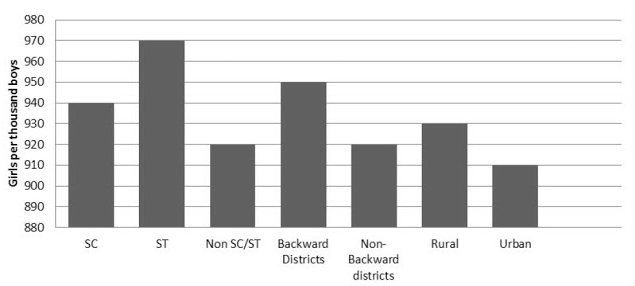(ii) From the above graph, we can conclude that the maximum number of girls per thousand boys is present in the section ST. We can also observe that the backward districts and rural areas have more number of girls per thousand boys than non-backward districts and urban areas.

Q3. Given below are the seats won by different political parties in the polling outcome of a state assembly elections: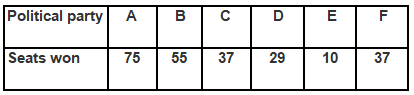(i) Draw a bar graph to represent the polling results.
(ii) Which political party won the maximum number of seats?
Ans:
(i) The bar graph representing the polling results is given below: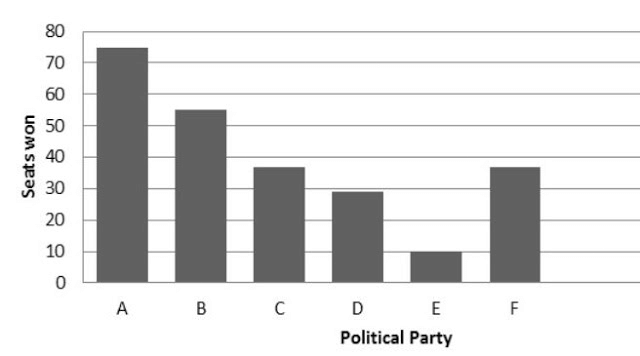(ii) From the bar graph it is clear that Party A won the maximum number of seats.

Q4. The length of 40 leaves of a plant are measured correct to one millimetre, and the obtained data is represented in the following table:
(i) Draw a histogram to represent the given data. [Hint: First make the class intervals continuous]
(ii) Is there any other suitable graphical representation for the same data?
(iii) Is it correct to conclude that the maximum number of leaves are 153 mm long? Why?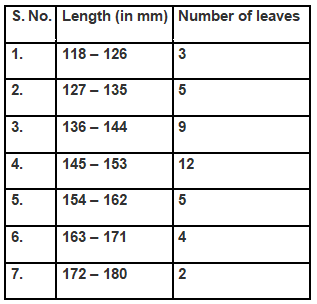Ans: (i) The data given in the question is represented in discontinuous class interval. So, we have to make it in continuous class interval. The difference is 1, so taking half of 1, we subtract ½ = 0.5 from lower limit and add 0.5 to the upper limit. Then the table becomes: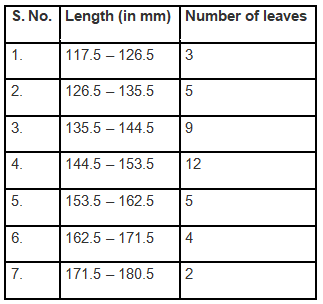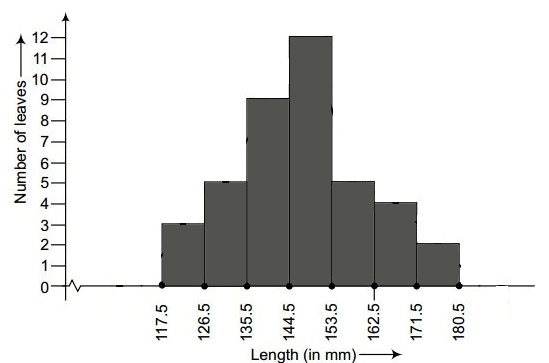(ii) Yes, the data given in the question can also be represented by frequency polygon.
No, we cannot conclude that the maximum number of leaves are 153 mm long because the maximum number of leaves are lying in-between the length of 144.5 – 153.5

Q5. The following table gives the life times of 400 neon lamps: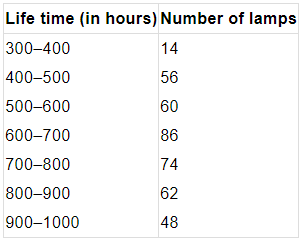(i) Represent the given information with the help of a histogram.
(ii) How many lamps have a life time of more than 700 hours?
Ans:
(i) The histogram representation of the given data is given below: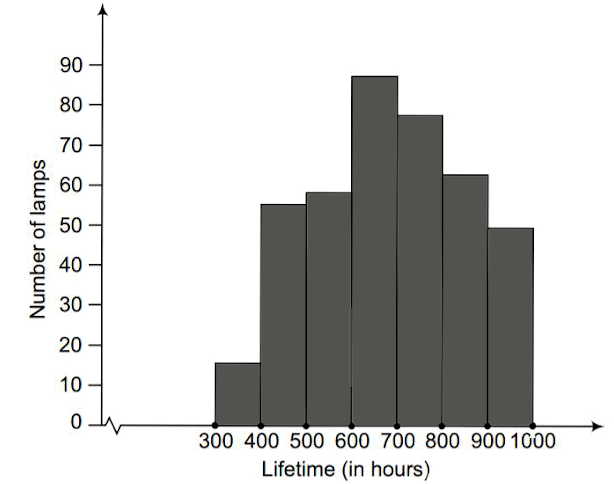(ii) Number of lamps having life time more than 700 hours = 74 + 62 + 48 = 184.

Q6. The following table gives the distribution of students of two sections according to the marks obtained by them: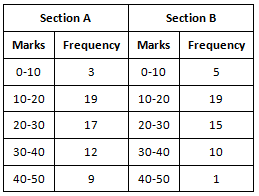Represent the marks of the students of both the sections on the same graph by two frequency polygons. From the two polygons compare the performance of the two sections.
Ans:
The class-marks = (lower limit + upper limit)/2
For section A,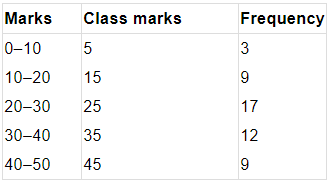For section B: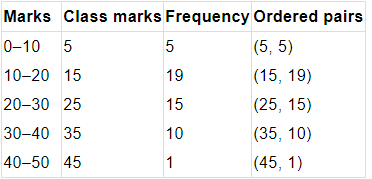Representing these data on a graph using two frequency polygon we get,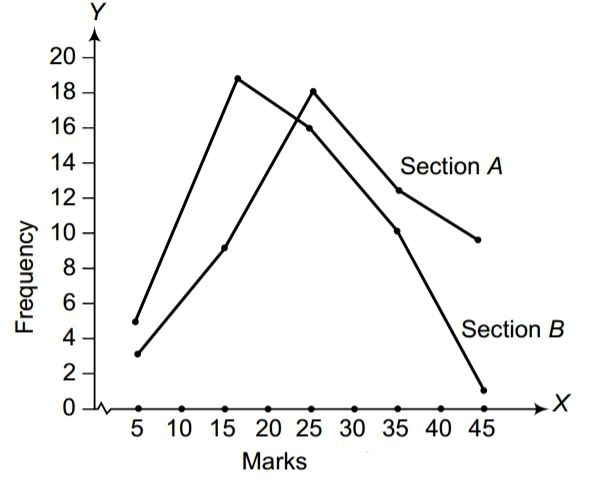From the graph, we can conclude that the students of Section A performed better than Section B.

Q7. The runs scored by two teams A and B on the first 60 balls in a cricket match are given below: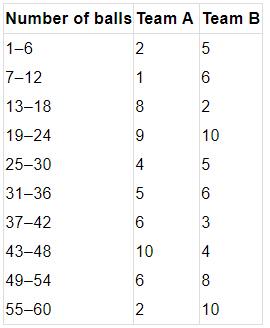Represent the data of both the teams on the same graph by frequency polygons.
Note: The given class intervals are not continuous. Therefore, we first modify the distribution as continuous.
Ans:
The data given in the question is represented in discontinuous class interval. So, we have to make it in continuous class interval. The difference is 1, so taking half of 1, we subtract ½ = 0.5 = 0.5 from lower limit and add 0.5 to the upper limit. Then the table becomes: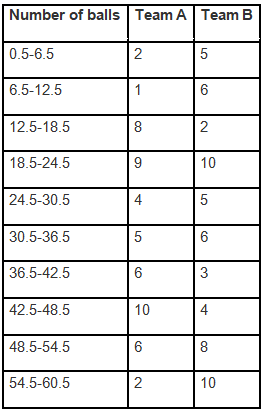The data of both the teams are represented on the graph below by frequency polygons.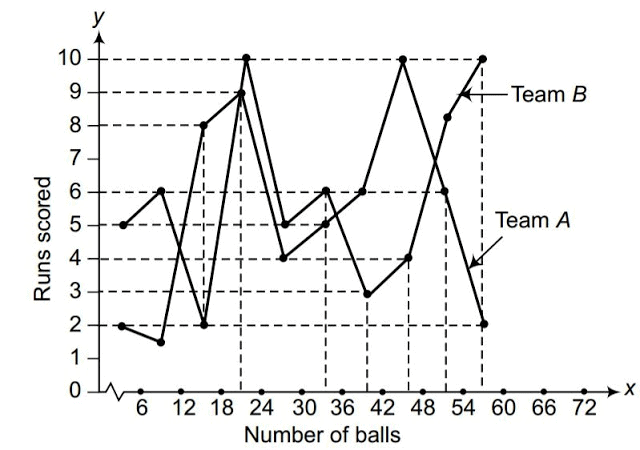Q8. A random survey of the number of children of various age groups playing in a park was found as follows: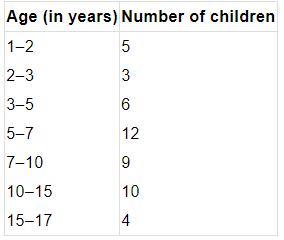Draw a histogram to represent the above data.
Ans:
The width of the class intervals in the given data is varying.
We know that,
The area of rectangle is proportional to the frequencies in the histogram.
Thus, the proportion of the children per year can be calculated as given in the table below.
Now, we draw the histogram taking ages (in years) on the x-axis and corresponding adjusted frequencies on the y-axis as shown below: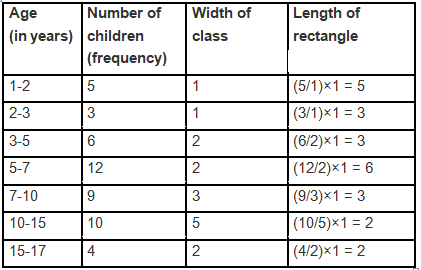Let x-axis = the age of children
y-axis = proportion of children per 1 year interval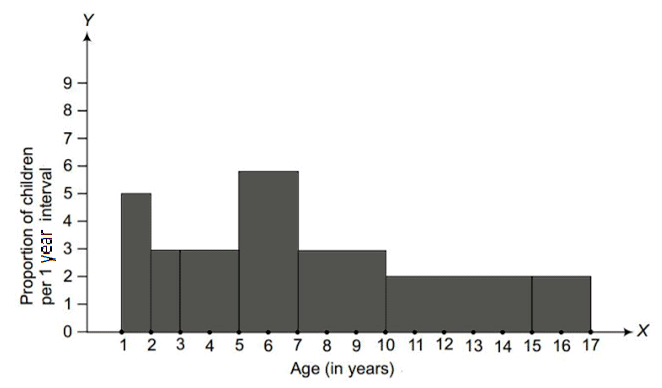Q9. 100 surnames were randomly picked up from a local telephone directory and a frequency distribution of the number of letters in the English alphabet in the surnames was found as follows: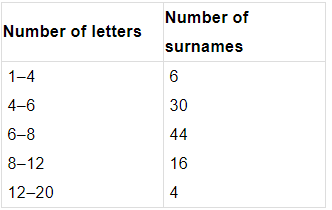(i) Draw a histogram to depict the given information.
(ii) Write the class interval in which the maximum number of surnames lie.

Ans:
(i) The width of the class intervals in the given data is varying.
We know that,
The area of rectangle is proportional to the frequencies in the histogram.
Thus, the proportion of the number of surnames per 2 letters interval can be calculated as given in the table below.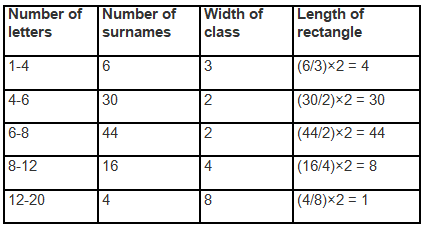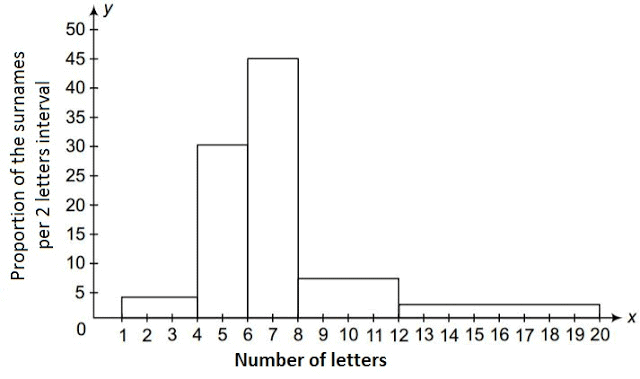(ii) 6-8 is the class interval in which the maximum number of surnames lie.

MEASURES OF CENTRAL TENDENCY

We can make out some important features of given data by considering only certain representatives.

These representatives are called the measures of central tendency or averages. There are three main averages: Mean, Median and Mode.
Mean The mean (or average) of a number of observations is the sum of the values of all the observations divided by the total number of observations. It is denoted by the symbol x and we read it as x-bar.
Thus, the mean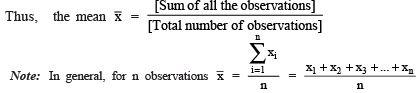Here, ∑ is a Greek symbol called sigma.
The summation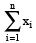is read as the sum of the x as i varies from 1 to n.

Mode

The mode is the value of the observation which occurs most frequently, i.e., an observation with the maximum frequency is called the mode of the data.
Note: In a given data, the value around which there is greatest concentration, is called the mode of the data.

Median After arranging the given data in an ascending or a descending order of magnitude, the value of the middle-most observation is called the median of the data.
Note: For ‘n’ observations (taken in order),

(i) if n is odd, the median = value of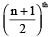observation.
(ii) if n is even, the median = mean of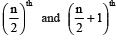observations.

The document Statistics (Exercise 12.1) NCERT Solutions | Mathematics (Maths) Class 9 is a part of the Class 9 Course Mathematics (Maths) Class 9.
All you need of Class 9 at this link: Class 9

## FAQs on Statistics (Exercise 12.1) NCERT Solutions - Mathematics (Maths) Class 9

 1. What is the importance of statistics in data analysis?Ans. Statistics plays a crucial role in data analysis as it helps in organizing, summarizing, and interpreting data. It provides valuable insights and patterns from large datasets, allowing us to make informed decisions based on evidence rather than intuition.
 2. How can statistics be used in making predictions?Ans. Statistics can be used to make predictions by analyzing past data and identifying patterns or trends. By using statistical techniques such as regression analysis, we can develop models that can predict future outcomes based on the relationships observed in the data.
 3. What are the different types of statistical measures used in data analysis?Ans. There are several types of statistical measures used in data analysis, including measures of central tendency (mean, median, mode), measures of dispersion (range, variance, standard deviation), and measures of association (correlation coefficient, regression coefficient).
 4. How can statistics help in making informed business decisions?Ans. Statistics can help in making informed business decisions by providing valuable insights into customer behavior, market trends, and performance analysis. By analyzing data using statistical techniques, businesses can identify areas of improvement, optimize operations, and make strategic decisions that maximize profitability.
 5. What are the limitations of statistics in data analysis?Ans. While statistics is a powerful tool in data analysis, it has some limitations. These include the potential for sampling errors, the reliance on assumptions, the possibility of outliers skewing results, and the inability to establish causation. It is important to be aware of these limitations and to interpret statistical results with caution.

## Mathematics (Maths) Class 9

62 videos|426 docs|102 tests

## Mathematics (Maths) Class 9

62 videos|426 docs|102 tests
Signup to see your scores go up within 7 days! Learn & Practice with 1000+ FREE Notes, Videos & Tests.
10M+ students study on EduRev
Track your progress, build streaks, highlight & save important lessons and more!(Scan QR code)
Related Searches

,

,

,

,

,

,

,

,

,

,

,

,

,

,

,

,

,

,

,

,

,

;